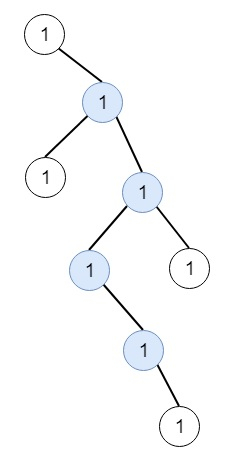# Longest ZigZag Path in a Binary Tree in C++

Suppose we have a binary tree root, a ZigZag path for a binary tree is defined as follow −

• Choose any node in the binary tree and a direction (either right or left).

• If the current direction is right then moving towards the right child of the current node otherwise move towards the left child.

• Then change the direction from right to left or vice versa.

• Repeat the second and third steps until we can't move in the tree.

Here the Zigzag length is defined as the number of nodes visited - 1. (A single node has a length of 0). We have to find the longest ZigZag path contained in that tree. So for example, if the tree is like −The output will be 3, as (Right, Left, Right)

To solve this, we will follow these steps −

• Define a method called dfs(), this will take root and leftB

• if root is null, then return -1

• if root is only one node in the tree, then return 0

• leftV := dfs(left of root, true) and rightV := dfs(right of root, false)

• ret := max of ret and (1 + max of leftV and rightV)

• if leftB is not 0, then return 1 + rightV, otherwise return 1 + leftV

• From the main method, set ret := 0

• call dfs(root, true) and call dfs(root, false)

• return ret

## Example (C++)

Let us see the following implementation to get a better understanding −

Live Demo

#include <bits/stdc++.h>
using namespace std;
class TreeNode{
public:
int val;
TreeNode *left, *right;
TreeNode(int data){
val = data;
left = right = NULL;
}
};
void insert(TreeNode **root, int val){
queue<TreeNode*> q;
q.push(*root);
while(q.size()){
TreeNode *temp = q.front();
q.pop();
if(!temp->left){
if(val != NULL)
temp->left = new TreeNode(val);
else
temp->left = new TreeNode(0);
return;
} else {
q.push(temp->left);
}
if(!temp->right){
if(val != NULL)
temp->right = new TreeNode(val);
else
temp->right = new TreeNode(0);
return;
}else{
q.push(temp->right);
}
}
}
TreeNode *make_tree(vector<int> v){
TreeNode *root = new TreeNode(v);
for(int i = 1; i<v.size(); i++){
insert(&root, v[i]);
}
return root;
}
class Solution {
public:
int ret;
int dfs(TreeNode* root, bool leftB){
if(!root) return -1;
if(!root->left && !root->right) return 0;
int leftV = dfs(root->left, true);
int rightV = dfs(root->right, false);
ret = max(ret, 1 + max(leftV, rightV));
if(leftB) return 1 + rightV;
return 1 + leftV;
}
int longestZigZag(TreeNode* root) {
ret = 0;
dfs(root, true);
dfs(root, false);
return ret;
}
};
main(){
vector<int> v = {1,NULL,1,1,1,NULL,NULL,1,1,NULL,1,NULL,NULL,NULL,1,NULL,1};
TreeNode *root = make_tree(v);
Solution ob;
cout << (ob.longestZigZag(root));
}

## Input

[1,null,1,1,1,null,null,1,1,null,1,null,null,null,1,null,1]

## Output

3